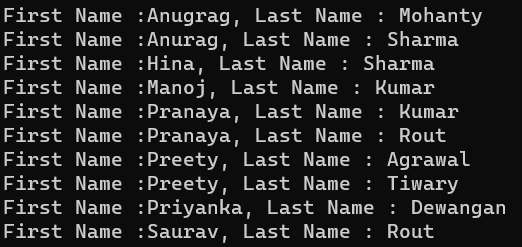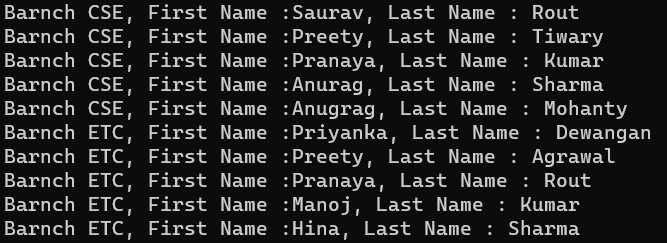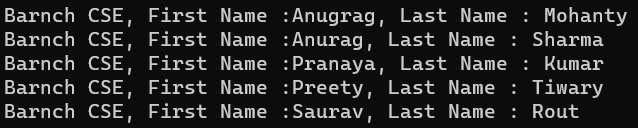# LINQ ThenBy and ThenByDescending Method in C#

## LINQ ThenBy and ThenByDescending Method in C# with Examples

In this article, I will discuss the LINQ ThenBy and ThenByDescending Method in C# with Examples. Please read our previous article discussing the LINQ OrderByDescending Method in C# with Examples. At the end of this article, you will understand the following pointers:

1. Why do we need the ThenBy and ThenByDescending Method in C#?
2. What are the ThenBy and ThenByDescending Methods in C#?
3. Example of LINQ ThenBy and ThenByDescending Method using Method and Query Syntax.
4. How do you use the ThenBy and ThenByDescending along with the Filtering method?
5. When to Use ThenBy and ThenByDescending Methods in C#?
##### LINQ ThenBy and ThenByDescending Method in C#:

The LINQ OrderBy and OrderByDescending method works fine when you want to sort the data based on a single value or expression. But when you want to sort the data based on multiple values or expressions, you need to use the LINQ ThenBy and ThenByDescending Method along with the OrderBy or OrderByDescending Method.

That means if you want to sort the data by multiple keys, the first key will be sorted by the OrderBy or OrderByDescending method. And then, from the second key onwards, the data will be sorted by ThenBy and ThenByDescending methods. You can use the ThenBy or ThenByDescending method more than once in the same LINQ query, but you can use the OrderBy or OrderByDescending methods only once in the same query.

The OrderBy or OrderByDescending method is generally used for primary sorting. The LINQ ThenBy or ThenByDescending Methods are used for secondary sorting. For example, sort the student by First Name and then sort the student by Last Name. In this case, the First Name needs to be sorted by the OrderBy or OrderByDescending method, and the Last Name needs to be sorted by the ThenBy or ThenByDescending method based on our requirements.

##### Examples to Understand LINQ ThenBy and ThenByDescending Methods in C#:

We will use ThenBy or ThenByDescending methods, so we need to work with Complex types. It is not possible to use these two methods with primitive data types as we are going to sort the data based on multiple keys. So, let us see how we can work with Complex data types using LINQ ThenBy or ThenByDescending methods. We will use the following Student class to understand the use of the ThenBy and ThenByDescending methods in C#. So, create a class file named Student.cs and copy and paste the following code.

```using System.Collections.Generic;
namespace LINQDemo
{
public class Student
{
public int ID { get; set; }
public string FirstName { get; set; }
public string LastName { get; set; }
public string Branch { get; set; }

public static List<Student> GetAllStudents()
{
List<Student> listStudents = new List<Student>()
{
new Student{ID= 101,FirstName = "Preety",LastName = "Tiwary",Branch = "CSE"},
new Student{ID= 102,FirstName = "Preety",LastName = "Agrawal",Branch = "ETC"},
new Student{ID= 103,FirstName = "Priyanka",LastName = "Dewangan",Branch = "ETC"},
new Student{ID= 104,FirstName = "Hina",LastName = "Sharma",Branch = "ETC"},
new Student{ID= 105,FirstName = "Anugrag",LastName = "Mohanty",Branch = "CSE"},
new Student{ID= 106,FirstName = "Anurag",LastName = "Sharma",Branch = "CSE"},
new Student{ID= 107,FirstName = "Pranaya",LastName = "Kumar",Branch = "CSE"},
new Student{ID= 108,FirstName = "Manoj",LastName = "Kumar",Branch = "ETC"},
new Student{ID= 109,FirstName = "Pranaya",LastName = "Rout",Branch = "ETC"},
new Student{ID= 110,FirstName = "Saurav",LastName = "Rout",Branch = "CSE"}
};

return listStudents;
}
}
}```

As you can see, we created the above Student class with four properties: ID, FirstName, LastName, and Brach. We then created one method, i.e., GetAllStudents(), which will return a list of students, which will be our data source, and on this data source, we will perform our operations.

##### Example to Understand LINQ ThenBy Method Using Method Syntax in C#:

Our requirement is to sort the student by First Name in Ascending Order, and then we need to sort the student by Last Name in Ascending order. So, here, we will sort the data based on two keys, i.e., FirstName and LastName. So, in this case, the first sorting will be done by the OrderBy method, and the second will be done by the ThenBy method, as shown in the example below.

```using System;
using System.Linq;
namespace LINQDemo
{
class Program
{
static void Main(string[] args)
{
//Sorting the Student data by First Name and LastName in Descending Order
//Using Method Syntax
var MS = Student.GetAllStudents()
.OrderBy(x => x.FirstName)
.ThenBy(y => y.LastName)
.ToList();

foreach (var student in MS)
{
Console.WriteLine("First Name :" + student.FirstName + ", Last Name : " + student.LastName);
}

}
}
}
```

Run the above application, and you will get the following output. Here, you can observe a few students, such as Anurag, Pranaya, and Preety, having the same First Name and different last names. For those students, first, it will sort them using their First Name, and then it will sort them using their Last Name, which you can see in the image below.##### Example to Understand LINQ ThenBy Method Using Query Syntax in C#

We do not have any operators called ThenBy and ThenByDescending, which we can use in the query syntax. So here, we need to specify multiple values or expressions in the order by clause separated by a comma, as shown in the below example.

```using System;
using System.Linq;
namespace LINQDemo
{
class Program
{
static void Main(string[] args)
{
//Sorting the Student data by First Name and Last Name in Descending Order
//Using Query Syntax
var QS = (from std in Student.GetAllStudents()
orderby std.FirstName, std.LastName
select std);

foreach (var student in QS)
{
Console.WriteLine("First Name :" + student.FirstName + ", Last Name : " + student.LastName);
}

}
}
}
```

Now, run the above code, which will also give you the same output as expected, as shown in the image below.##### Example to Understand Ascending and Descending with Multiple Key Values:

Let us see an example of how we can Perform the Ascending and Descending Operation with Multiple Key Values using Method and Query Syntax in C#. Our requirement is that we need to first sort the students in ascending order based on the Branch. Then, we must sort the students in descending order based on their First Names. And finally, we need to sort the students in ascending order based on their Last Names. The following example exactly does the same.

```using System;
using System.Linq;
namespace LINQDemo
{
class Program
{
static void Main(string[] args)
{
//First Sort Students in Ascending Order Based on Branch
//Then Sort Students in Descending Order Based on FirstName
//Finally Sort Students in Ascending Order Based on LastName

//Using Method Syntax
var MS = Student.GetAllStudents()
.OrderBy(x => x.Branch)
.ThenByDescending(y => y.FirstName)
.ThenBy(z => z.LastName)
.ToList();

//Using Query Syntax
var QS = (from std in Student.GetAllStudents()
orderby std.Branch ascending,
std.FirstName descending,
std.LastName //by default ascending
select std).ToList();

foreach (var student in QS)
{
Console.WriteLine("Barnch " + student.Branch + ", First Name :" + student.FirstName + ", Last Name : " + student.LastName);
}

}
}
}```

Now, run the application, and you will get the following output as expected.##### Key Points to Rember:

ThenBy: The LINQ ThenBy Method is used for secondary sorting in ascending order. It’s often used after OrderBy when sorting the collection on another criterion if the primary key is identical.

ThenByDescending: The LINQ ThenByDescending method is similar to ThenBy but sorts the collection in descending order for the secondary key.

• ThenBy and ThenByDescending can only be used after an OrderBy or OrderByDescending method. They cannot be used as standalone methods.
• These methods are used for multi-level sorting – first by one property and then another.
• ThenBy and ThenByDescending use deferred execution.
• They can be chained multiple times for additional sorting criteria.
##### Example Using ThenBy and ThenByDescending Method with Where Extension Method:

Let us see an example to understand how we can use LINQ ThenBy and ThenByDescending Method with Where Extension Method in C#. Our requirement is first, we need to fetch only the CSE branch students, and then we need to sort the data as follows.

1. First, sort the students in ascending order based on First Name.
2. Then, sort the students in descending order based on their Last Names.

The following example code exactly does the same.

```using System;
using System.Linq;
namespace LINQDemo
{
class Program
{
static void Main(string[] args)
{
//First, fetch only the CSE branch students
//Sort the Students in ascending order based on First Name
//Sort the students in descending order based on their Last Names

//Using Method Syntax
var MS = Student.GetAllStudents()
.Where(std => std.Branch == "CSE")
.OrderBy(x => x.FirstName)
.ThenByDescending(y => y.LastName)
.ToList();

//Using Query Syntax
var QS = (from std in Student.GetAllStudents()
where std.Branch == "CSE"
orderby std.FirstName, //By Default it is Ascending
std.LastName descending
select std).ToList();

foreach (var student in QS)
{
Console.WriteLine("Barnch " + student.Branch + ", First Name :" + student.FirstName + ", Last Name : " + student.LastName);
}

}
}
}```

Now, run the application, and you will get the following output as expected.##### When to Use LINQ ThenBy and ThenByDescending Method in C#?

Here are some typical use cases:

• Multi-level Sorting in Data Display: When presenting data to users, especially in tables or lists, you might first sort by one key (like a category or a date) and then by another (like a name or a value). For instance, displaying products is sorted by category and price.
• Reports and Data Analysis: In generating reports or performing data analysis, ThenBy and ThenByDescending are used to sort data by primary and secondary criteria. For example, sorting sales data first by region and then by the sales amount.
• Organizing Data for Better Readability: Secondary sorting makes the data more readable and logical. For instance, sorting a list of employees by department and alphabetically by their names.
• Creating Hierarchical Sort Orders: In scenarios where data needs to be sorted hierarchically, like sorting a file directory first by file type and then by file name or size.

In the next article, I will discuss the LINQ Reverse Method in C# with Examples. I hope this article gives you a very good understanding of how and when to use the LINQ ThenBy and ThenByDescending Method in C# with Examples. I hope you enjoy this article.